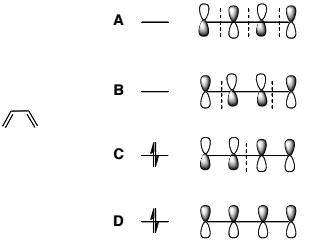Problem: Consider the Molecular Orbitals (MO's) of butadiene. Which are the HOMO and LUMO? 1) HOMO = B, LUMO = C 2) HOMO = D, LUMO = A 3) HOMO = C, LUMO = B 4) HOMO = A, LUMO = D 5) HOMO = D, LUMO = B

FREE Expert Solution
79% (350 ratings)
Problem Details

Consider the Molecular Orbitals (MO's) of butadiene. Which are the HOMO and LUMO?

1) HOMO = B, LUMO = C

2) HOMO = D, LUMO = A

3) HOMO = C, LUMO = B

4) HOMO = A, LUMO = D

5) HOMO = D, LUMO = BWhat scientific concept do you need to know in order to solve this problem?

Our tutors have indicated that to solve this problem you will need to apply the HOMO LUMO concept. You can view video lessons to learn HOMO LUMO Or if you need more HOMO LUMO practice, you can also practice HOMO LUMO practice problems .

What is the difficulty of this problem?

Our tutors rated the difficulty of Consider the Molecular Orbitals (MO's) of butadiene. Which a... as medium difficulty.

How long does this problem take to solve?

Our expert Organic tutor, Johnny took 3 minutes to solve this problem. You can follow their steps in the video explanation above.

What professor is this problem relevant for?

Based on our data, we think this problem is relevant for Professor Carrico's class at SUNY.